# Motor powers, losses and returns

Subjects:

• Indicated power (Pi)
• Effective power (Pe)
• ISO power

Indicated power (Pi)
The power generated by combustion is called the indicated power. The English translation of “indicated power” is Indicated Mean Effective Pressure, abbreviated as: IMEP. This internal power is determined from the indicator diagram.

The same indicator diagram can be seen in the images below. The right one is a symbol for calculating the areas: the positive (blue) and the negative area (green). When we have determined the relative surface (positive minus negative) we speak of the average combustion pressure. In reality, this is calculated mathematically by means of integral calculus.

With the indicated power we look at the average surface in the indicator diagram. The power measured at the crankshaft will be lower due to mechanical losses.

Effective Power (Pe):
The effective power (in English: Brake Mean Effective Pressure, abbreviated as BMEP) is the power that is left after the friction losses of the indicated power.

With this formula we are going to calculate the power that the engine delivers to its flywheel at the speed at which the torque is highest. The effective power will be lower than the indicated power because there are friction losses that occur from the piston to the wheels, and auxiliary units such as the oil pump, coolant pump and alternator absorb power while the engine is running. The formula is: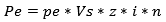In which:

• Pe = total power supplied;
• pe = mean effective pressure;
• Vs = stroke volume;
• z = number of cylinders;
• i = 1 for two-stroke and 0,5 for four-stroke;
• n = speed.

We assume the following data for the calculation:

• Average effective piston pressure: 1400000 N/m² (= 14 bar);
• Displacement volume: 1,59 dm³ (0,3975 dm³ per cylinder);
• Number of cylinders: 4
• 4-stroke
• Speed: 3000 rpm.

Here is the calculation again:We fill in the data and do not take scientific notations into account for the time being.

Important: we convert the swept volume per cylinder from dm³ to m³ (divide by 1000, see: Calculate content). 0,3975 dm³ then becomes: 0,0003975 m³.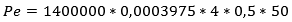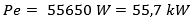It follows that the motor delivers a power of 55,7 kW at the given speed.

In the previous example, the mean effective pressure (pe) was given and the power delivered (Pe) was requested. However, we cannot easily determine pe. After all, there is no measuring equipment for this. For a given Pe and unknown pe, we can still find out by converting the formula. We will do this in the following example.

Data from an engine:

• Maximum power: 147 kW at 5700 rpm;
• Bore: 82.5mm;
• Stroke: 92.8 mm

We fill in the data in the formula: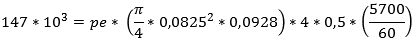We convert the formula so that the unknown is placed to the left of the comparison sign: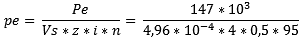We convert the result to the pressure in bar: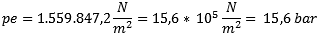ISO power:
The ISO power is determined at the engine test stand, where the manufacturer runs the engine under the following operating conditions:

• outside air pressure (p) = 1 bar;
• air temperature (T) = 300 K = 26,85 degrees Celsius;
• relative humidity (RH) = 60%
• coolant temperature inlet air cooler (Ti) = 300 K = 26,85 degrees Celsius;
• Lower calorific value / heating value (H0) = 42 MJ/kg.

Related page:error: Alert: Content is protected !!